AP Economics

Mr. Crawford

Semester Exam Review Guide

Structure:

60 Multiple Choice questions (1 point each) in 70 minutes

3 Essays (20 points) in 60 minutes

UNIT ONE
FUNDAMENTALS OF ECONOMICS
• CHOICE
• EUSR=MSUW
• OPPORTUNITY COST
• TINSTAAFL
• SCARCITY
• LIMITED AND DESIRABLE
• RATIONAL THINKING
• COST-BENEFIT ANALYSIS
• FUTURE UTILITY
• INVESTMENT
• MARGINAL THINKING
• IMPERFECT INFORMATION
• ECONOMIC THEORY
• POSITIVE/OBJECTIVE
• FACTS
• THEORY
• INDUCTION
• DEDUCTION
• NORMATIVE/SUBJECTIVE
• POLICY
• FALLACIES
• POST HOC
• COMPOSITION
• DEFINITION
• BIAS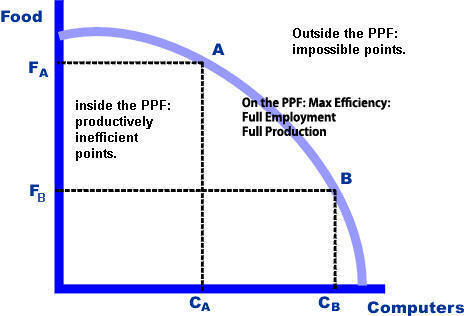UNIT TWO
THE MARKET SYSTEM
• THE FOUR FUNDAMENTAL QUESTIONS
• BARTER

• GREED
• PROFIT MOTIVE
• PRIVATE PROPERTY
• FREEDOM OF CHOICE
• MARKETS
• COMPETITION
• NO GOVT
• "LAISSEZ FAIRE"
• "INVISIBLE HAND"
• CAPITALISM
• SPECIALIZATION
• CAPITAL
• MONEY
• CONSUMER SOVEREIGNTY
• DERIVED DEMAND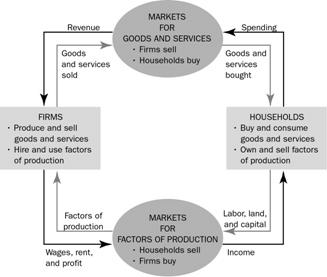UNIT THREE
SUPPLY AND DEMAND
DEMAND

Law of Demand

CHANGE IN P= CHANGE IN Qd

INVERSE

• DMU
• YFX
• SFX

CHANGE IN NPD= CHANGE IN D

• TASTES
• INCOME
• EXPECTATIONS
• RELATED GOODS' PRICES
• SUB=SAME
• COMP=OPPOSITE

CHANGE IN D= PQCHANGE IN D= PQ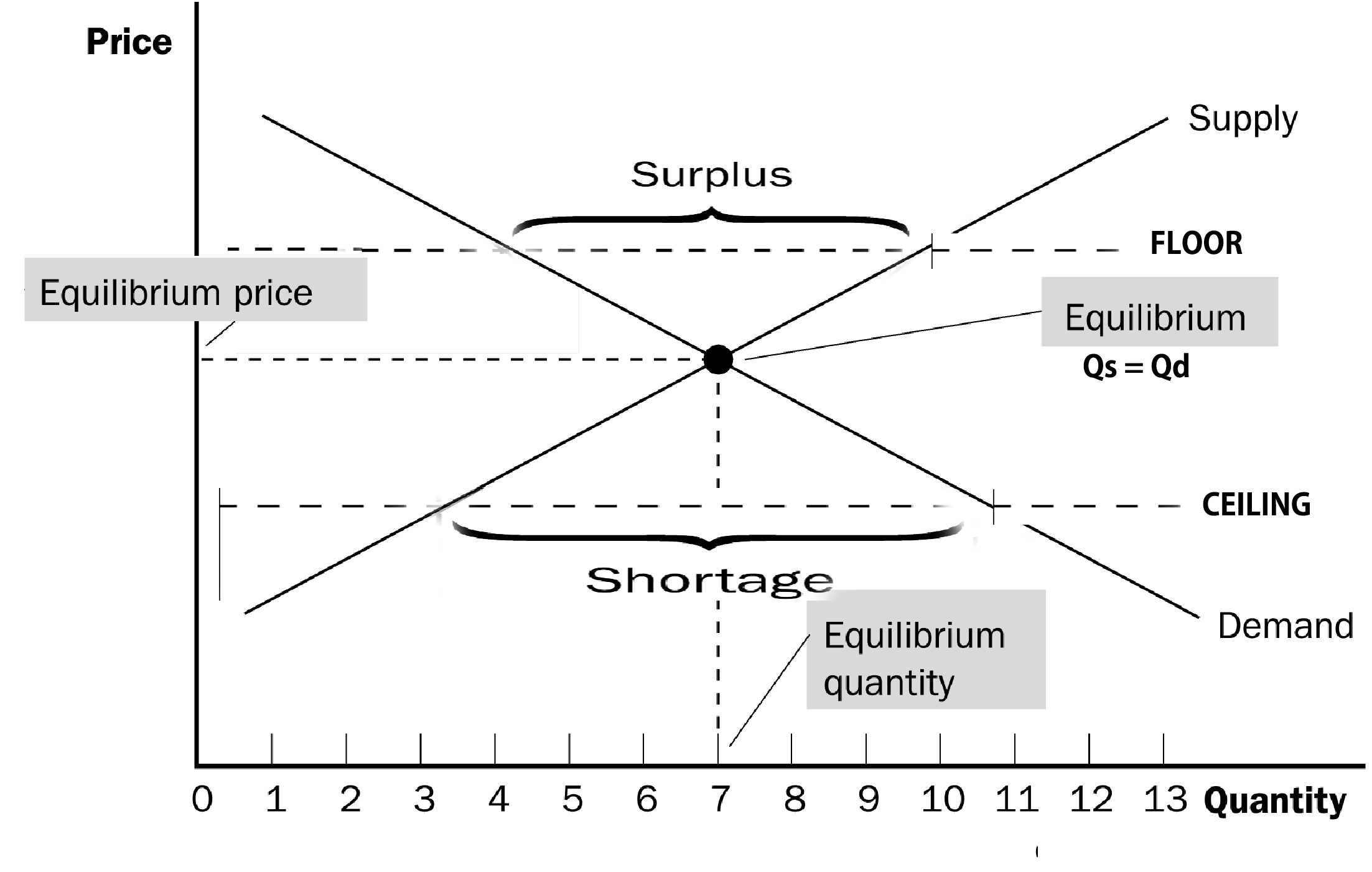SUPPLY

Law of Supply

CHANGE IN P =  CHANGE IN Qs

DIRECT

• DMR/IC
• P X Q = TR

CHANGE IN NPD= CHANGE IN S

• GOVERNMENT
• TAXES/SUBSIDIES
• PRICE CONTROLS
• OTHER PROFIT OPPORTUNITIES
• NUMBER
• INVESTMENT IN TECHNOLOGY
• COST OF RESOURCE
• EXPECTATIONS

CHANGE IN S= PQCHANGE IN S= PQUNIT FOUR
MARKET FAILURE
KARL MARX
• WEALTH = UTILITY
• LABOR THEORY OF VALUE
• ALIENATION
• EXPLOITATION
• SUBSISTENCE
• PROFIT IS SURPLUS LABOR
• FLAWS OF CAPITALISM
• EXTERNALITIES
• PUBLIC COSTS
• ASYMMETRIC INFORMATION
• PUBLIC GOODS
• IMPERFECT COMPETITION
• MONOPOLY
• INEQUITY
• SOLUTION
• UNIONS
• REVOLUTION
• GOVERNMENT
• PUBLIC OWNERSHIP

SOCIALISMUNIT FIVE
MACROECONOMIC INSTABILITY

• SAYS' LAW:  Y = C

• MARX: INHERENT INSTABILITY BECAUSE

• Y = C + S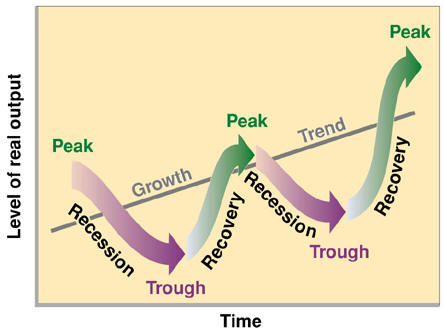AGGREGATE DEMAND

Law of Aggregate Demand

CHANGE IN PL = CHANGE IN NATIONAL OUTPUT (GDP, EMPLOYMENT, NATIONAL INCOME)

INVERSE

• FOREIGN PURCHASES EFFECT
• WEALTH EFFECT
• INTEREST RATE EFFECT

CHANGE IN NPD= CHANGE IN AD

• CONSUMER DEBT
• REAL WEALTH
• CONSUMER EXPECTATIONS

CHANGE IN AD= PLAQCHANGE IN AD= PLQLSHORT RUN AGGREGATE SUPPLY

Law of Aggregate Supply

CHANGE IN PL =  CHANGE IN CHANGE IN NATIONAL OUTPUT (GDP, EMPLOYMENT, NATIONAL INCOME)

DIRECT

CHANGE IN NPD= CHANGE IN AS

• GOVERNMENT
• TAXES/SUBSIDIES
• EXPECTATIONS
• COST OF RESOURCE

CHANGE IN AS= PLAQCHANGE IN AS= PLAQ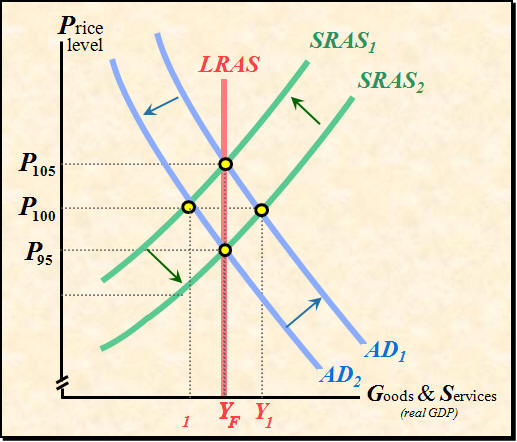LONG RUN AGGREGATE SUPPLY

LRAS = PPF

PERFECTLY INELASTIC; UNRELATED TO CHANGES IN PL, LIMITS OF POTENTIAL PRODUCTION AT FULL EMPLOYMENT

CHANGE IN NPD= CHANGE IN LRAS

• RESOURCES
• TECHNOLOGY

CLASSICAL THEORY

• JOSEPH SCHUMPETER
• Y = C + S
• S = I
• Y = C + I
• CYCLES ARE TEMPORARY DISEQUILIBRIUMS IN
• PRODUCT MARKET
• RESOURCE MARKET
• MONEY MARKET
• IF ADTHEN S > I
• SURPLUS; RECESSION
• PRICES DROP
• DEFLATION OR DISINFLATION
• WAGES DROP
• INTEREST RATES DROP
• ASAND S = I
• IF ADTHEN S < I
• SHORTAGE; INFLATION
• PRICES RISE
• INFLATION
• WAGES RISE
• INTEREST RATES RISE
• ASAND S = I
UNIT SIX
KEYNESIANISM
 CRITIQUES OF CLASSICAL THEORY SAVINGS DOES NOT EQUAL INVESTMENT RATCHET EFFECT DOWNWARD INFLEXIBILITY OF PRICES AND WAGES KEYNESIAN CONSUMPTION MULTIPLIER KCM = 1/MPS NATIONAL INCOME ACCOUNTING C + Ig + G + Xn = GDP NY + KCA + IBT = GNP CONSUMER PRICE INDEX NOMINAL = PxQ REAL = NOMINAL/CPI x 100 MARKET BASKET = HOLD QUANTITY CONSTANT CPI = MARKET BASKET OF THIS YEAR/MARKET BASKET OF BASE YEAR x 100 UNEMPLOYMENT LABOR POOL + NOT COUNTED = POPULATION UNEMPLOYMENT RATE = UNEMPLOYED/LABOR POOL UNEMPLOYMENT: ACTUAL FRICTIONAL CYCLICAL STRUCTURAL OKUN'S LAW CYCLICAL UNEMPLOYMENT x 2.5 = GDP GAP

COST

Aggregate Demand and Equilibrium
A. Equilibrium (E) is where planned and actual AD and AS are equal.
1. Equilibrium is where all goods produced for sale are sold.
2. At points below equilibrium, AD < AS, inventories are building and business
activity is contracting. This level of economic activity was depicted by the
horizontal (Keynesian) range of AS explained in the previous model.
3. At points above equilibrium AD > AS, inventories are decreasing and
as depicted by the intermediate range and eventually the classical range of AS.
4. Economic activity (Real GDP) will be wherever AD intersects AS.
Equilibrium seldom exists as
economic activity is usually
in one stage or another of the business cycle.
B. If economic activity is not in balance, a dynamic situation
exists and will continue
until equilibrium is reached.

C. Keynes believed that E could settle at a level of economic activity with large amounts of unemployment.
1. If potential Real GDP is greater than what actual AD yields, a recessionary gap exists and may persist indefinitely. The solution to this unacceptable
2. If potential Real GDP is less than what actual AD yields, an inflationary gap exists and the inflation may persist indefinitely. The solution to this unacceptable level of economic activity is to decrease AD.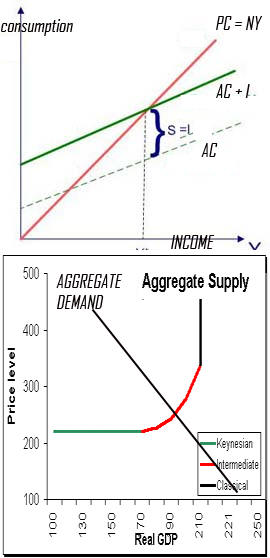MONETARY POLICY
MONETARY POLICY/SUPPLY SIDE
• PERMANENT INCOME THEORY
• EXPANSIONARY BIAS
• CROWDING OUT
• STRUCTURAL DEBT
• EXPORT SHOCK

EQUATION OF EXCHANGE

MV = PQ

FEDERAL RESERVE MONETARY TOOLS

• OPEN MARKET
• BOND PRICES
• DISCOUNT RATE
• DISCOUNT WINDOW
• RESERVE RATION
• HAMMER
• CHANGES BOTH M AND V
• LONG RUN
• IF EP > 0
• FIRMS ENTER
• ATC• P• EP = 0
• IF EP < 0
• FIRMS LEAVE
• ATC• P• EP = 0
• LONG RUN PRODUCTIVE EFFICIENCY
• X-EFFICIENCY
• OVERCAPITALIZATION FOR POTENTIAL EXPANSION
• NOT ALLOCATIVE EFFICIENT